# Microeconomics Cost Curve Analysis

2192 words (9 pages) Essay

9th Oct 2017 Economics Reference this

Disclaimer: This work has been submitted by a university student. This is not an example of the work produced by our Essay Writing Service. You can view samples of our professional work here.

Any opinions, findings, conclusions or recommendations expressed in this material are those of the authors and do not necessarily reflect the views of UKEssays.com.

Micro-economic Assignment

Question 1: Sketch the following cost curves and discuss the reasons for the shapes of the curves

1. The Marginal Cost Curve
2. The Average Cost Curve
3. The Average Fixed Cost Curve
4. The Average Variable Cost Curve

Costs at Julie Johnson’s photocopying store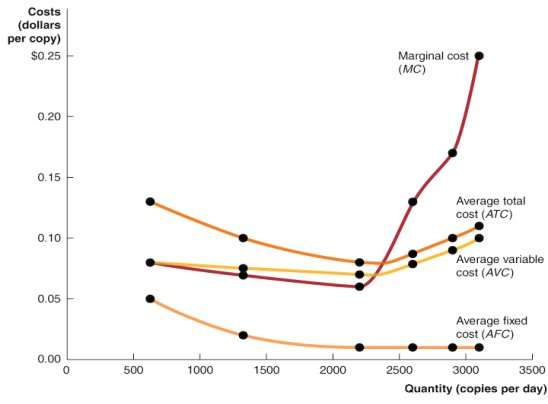• Diminishing returns applies only to the short run, when at least one of the firm’s inputs, such as the quantity of machinery it uses is fixed. The law of diminishing returns tells us that in the short run hiring more workers will, at some point, result in less additional output. Diminishing returns explains why marginal cost curves eventually slope upward.
• Diseconomices of scales apply in the long run, when the firm is free to vary al its inputs, can adopt new technology and can vary the amount of machinery it uses and the size of its facility. Diseconomies of scale explain why long run average cost curves eventually slope upwards.

Long run average cost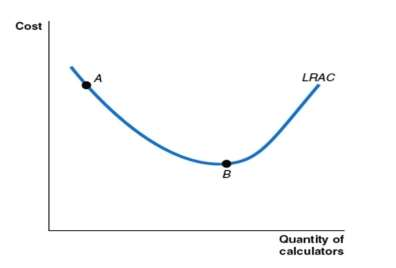The relationship between marginal cost and average total cost will explain why he average total cost curve also has a U-shape.

• It follows the usual relationship between marginal and average values.
• As long as marginal cost is below average total cost, average total cost will fall.
• When marginal cost is above average total cost, average total cost will rise.
• Marginal cost will equal average total cost when average total cost is at its lowest point

There are 3 key facts to understand to graphs those curves

1/ The marginal cost (MG), average total cost (ATC) and average variable cost (AVC) curves are all U-shaped, and the marginal cost curve intersects the average variable cost and average total cost curves at their minimum points. When marginal cost is less than either average variable cost or average variable cost, it causes them to decrease. When marginal cost it above average cost or average total cost, it causes them to increase. Therefore, when marginal cost equal average variable cost or average total cost, they must be at their minimum point.

If you need assistance with writing your essay, our professional essay writing service is here to help!

2/ as output increases, average fixed cost gets smaller and smaller. This happens because in calculating average fixed cost we are dividing something that gets larger and larger output into something that remains constant fixed cost. Firms often refer to this process of lowering average fixed cost by selling more output as ‘spreading the overhead’ where overhead refers to fixed cost.

3/ as output increases, the difference between average total cost and average variable cost decreases. This happens because the difference between average total cost and average variable cost is average fixed cost, which gets smaller as output increases

Question 2

For both perfect competition and monopolistic competition discuss with the use of diagrams, how profits are determined in the short-run and the long run. Outline the reasons for these outcomes

• Perfectly competitive markets is a market that meet the conditions of

2/ All firms selling identical products

3/ No barriers to new firms entering the market.

• A perfectly competitive cannot affect the market price because the market supply curve will not shift by enough to change the equilibrium price by even one cent.
• In perfectly competitive market, price is determined by the intersection of market and market supply. And if the demand curve is a horizontal line, it is perfect elastic. The profit is the different between Total revenue minus total cost. So, to maximize the profit, the firm should produce the quantity where the different between the total revenue receives and total cost is as large as possible. The profit-maximising level of output is also where marginal revenue equals marginal cost.
• If the price greater than average total cost, the firm will make a profit. If the price equal to average total cost the firm breaks even which mean the firm’s total cost equal its total revenue. If the price is smaller than the average total cost the firm will make a losses.
•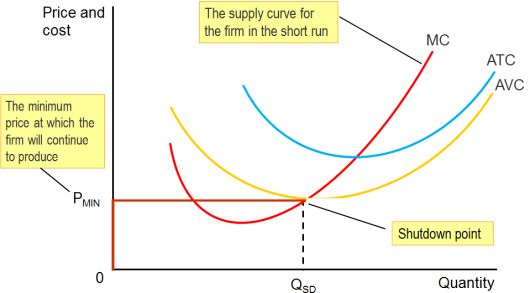The firm’s short-run supply curve
• In many case, the firm experiencing loses will consider stopping production temporarily. A firm can reduce its loss below the amount of its total fixed cost by continuing to produce provided that the total revenue it receives is greater than its variable cost. The revenue over and above variable cost can be used to cover part of the firm’s fixed cost. As a result, in this case the firm will have a smaller loss by continuing to produce than if it shut down. At lease they can survive with their business. But if the firm cannot recover its fixed cost by shutting down, those funds have been spent and cannot be recovered, so the firm should treat its sunk costs as less relevant to its decision making. Therefore, the firm’s total revenue is greater or less than its variable costs is the key to deciding whether or not to shut down. If the firm did raise its price it would lose all its customers and its sales would drop to zero.

If the price drops below average variable cost the firm will have a smaller loss if it shuts down and produces no output. So the firm’s marginal cost curve is its supply curve only for prices at or above average variable cost.

According to the graph above can give the conclusion in perfectly market competition, a firm maximize profit at the level of output at which marginal revenue equal to marginal cost. The difference between price and average total cost equal profit per unit of output. However, economic profit in a perfectly competitive industry is only a short run occurrence. As long as price is above average variable cost the firm will continue to produce in the short run, even they suffering losses. But it the price fall below the average variable cost the firm will shuts down, and the cannot suffering losses in long term.

In the long run, a perfectly competitive market will supply whatever amount of a good consumers demand at a price determined by the minimum point on the typical firm’s average total cost curve

The long-run supply curve in a perfectly competitive industry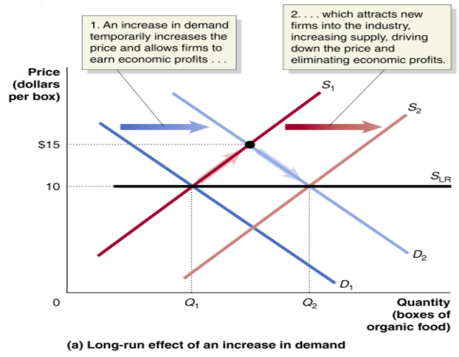• Monopoly: The only seller of a good or service which does not have a close substitute. A monopoly will produce less and charge a higher price than would a perfectly competitive industry producing the same good.
• Profit-maximising quantity and price for a monopoly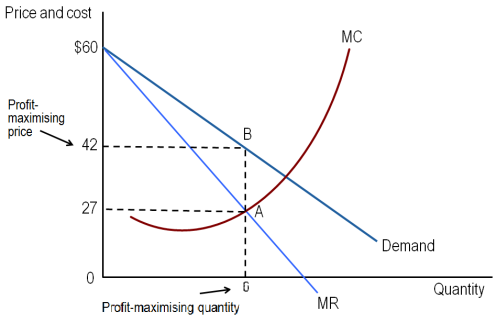• We assume that the firm’s marginal cost and average total cost curves have the usual U-shapes. To calculate its profit maximizing quantity and price, the marginal cost of selling one more subscription is less than the marginal revenue, the firm should sell additional subscription because it is adding to its profits. We can see in the graph showing that to maximize profit Austar, should sell subscription up to the point where the marginal revenue from selling the last subscription equals its marginal cost- point A. in this case the marginal revenue from selling the sixth subscription and the marginal cost are both \$27. Austar maximizes profit by selling six subscriptions per month and charging a price of \$42- point B. Its mean that the firm will maximize profit by selling subscriptions up to the point where marginal cost equal to marginal revenue.
• Additional, the tax is a fixed cost, no matter how many subscription it sells. Because the tax has no impact on firm’s marginal revenue or marginal cost, the profit maximizing level of output has not changed.
• Profits for a monopoly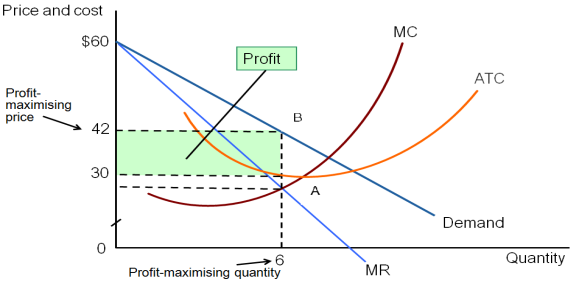This graph showing the green box represents Austar profit by selling six subscriptions per month and charging a price of \$42 minus the average total cost of \$30 and the base equal to the quantity of six subscriptions. Austar’s profit there for equal \$12 x 6 =\$72

Our academic experts are ready and waiting to assist with any writing project you may have. From simple essay plans, through to full dissertations, you can guarantee we have a service perfectly matched to your needs.

Even though Austar is earning economies profits new firms will not enter the market. Because Austar has a monopoly it will not face competition from other firm. Therefore, if other factors remain unchanged, Austar will be able to continue to earn economic profits, even in the long run.

Question 3

In what ways could a monopoly be (a) more efficient (b) less efficient than several firms competing against each other? Discuss this statement with the use of appropriate diag

The effect of monopoly as follows:

1/ monopoly causes a reduction in consumer surplus.

2/ monopoly causes an increase in producer surplus.

3/ monopoly causes a deadweight loss, which represents a reduction in economic effcuency, specifically a reduction in allocative efficiency.

A monopoly would be more efficient when it produce less and charge higher price than would a perfectly competitive industry producing the same good. This means that monopoly leads to allocative inefficiency that occurs when production reflects consumer preferences, and in particular production continues to pount where the price paid is equal to the marginal cost of production. Unlike perfect competition, a monopoly restricts output levels and charges a price higher than marginal cost.

Monopoly affect consumers producers and the efficiency of the economy. We measure consumer surplus as the area below the demand curve and above the market price. The higher the price is the smaller the consumer surplus. Because a monopoly raise the market price it reduces consumer surplus.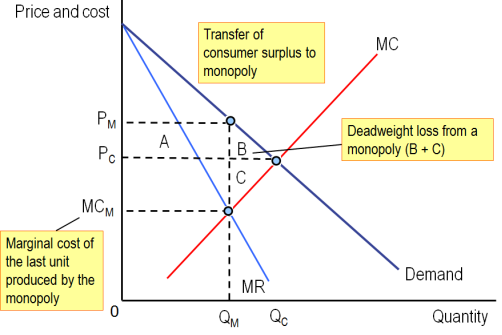In this diagram showing us that the loss of consumer surplus is equal to rectangle A plus triangle B. we measure the producer surplus as the area above the supply curve and below the market price. The increase in price due to monopoly increases producer surplus by an amount equal to rectangle A and reduces it by an amount equal to triangle C. because rectangle A is larger than triangle C, we know that a monopoly increase producer surplus compared with perfect competition.

Many firm, though, have market power which is ability of a firm to charge a price greater than marginal cost. The only firm that do not have market power are firm in perfectly competitive market, who must charge a price equal to marginal cost. Because few markets are perfectly competitive, some loss of economic efficiency occurs in the market for nearly every good and service. The closer price is to marginal cost the smaller the size of the deadweight loss. This is one of the main reasons why governments of market economies usually have policies promoting competition in the market.

The federal government has formed the ACCC to regulate business mergers because it knows that if firms agin market power by merging, they may use that market powerto raise prices and reduce output. The governmant is concerned with horizontal mergers or mergers between firms in the same industry. Vertical mergers which are merges between firms at dfferent stages of production of a good. As we seen in the graph above, it’s a result of this merger prices will rise and ouput will fall, leading to a decline in consumer surplus and economic efficiency.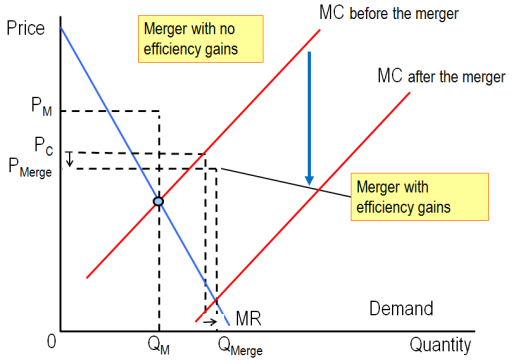In this graph the price declines after the merge from Pc to Pmerge and quantity increase from Qc to Qmerge. This lead to the following seemingly paradosical result: although the newly merged firm has a great deal of market power, because it is more efficient consumers are better off and economic efficiency is improved. Even if merged frim is more efficient and has lower costs, this may not offset the increased market power of the firm enouggh to increase consumer surplus and economic efficiency.

In the long run, the owners of the monopoly will not continue in business if they are experiencing losses, government regulatord set a price equal to average total cost.

Micro-economic Assignment

Question 1: Sketch the following cost curves and discuss the reasons for the shapes of the curves

1. The Marginal Cost Curve
2. The Average Cost Curve
3. The Average Fixed Cost Curve
4. The Average Variable Cost Curve

Costs at Julie Johnson’s photocopying store• Diminishing returns applies only to the short run, when at least one of the firm’s inputs, such as the quantity of machinery it uses is fixed. The law of diminishing returns tells us that in the short run hiring more workers will, at some point, result in less additional output. Diminishing returns explains why marginal cost curves eventually slope upward.
• Diseconomices of scales apply in the long run, when the firm is free to vary al its inputs, can adopt new technology and can vary the amount of machinery it uses and the size of its facility. Diseconomies of scale explain why long run average cost curves eventually slope upwards.

Long run average costThe relationship between marginal cost and average total cost will explain why he average total cost curve also has a U-shape.

• It follows the usual relationship between marginal and average values.
• As long as marginal cost is below average total cost, average total cost will fall.
• When marginal cost is above average total cost, average total cost will rise.
• Marginal cost will equal average total cost when average total cost is at its lowest point

There are 3 key facts to understand to graphs those curves

1/ The marginal cost (MG), average total cost (ATC) and average variable cost (AVC) curves are all U-shaped, and the marginal cost curve intersects the average variable cost and average total cost curves at their minimum points. When marginal cost is less than either average variable cost or average variable cost, it causes them to decrease. When marginal cost it above average cost or average total cost, it causes them to increase. Therefore, when marginal cost equal average variable cost or average total cost, they must be at their minimum point.

2/ as output increases, average fixed cost gets smaller and smaller. This happens because in calculating average fixed cost we are dividing something that gets larger and larger output into something that remains constant fixed cost. Firms often refer to this process of lowering average fixed cost by selling more output as ‘spreading the overhead’ where overhead refers to fixed cost.

3/ as output increases, the difference between average total cost and average variable cost decreases. This happens because the difference between average total cost and average variable cost is average fixed cost, which gets smaller as output increases

Question 2

For both perfect competition and monopolistic competition discuss with the use of diagrams, how profits are determined in the short-run and the long run. Outline the reasons for these outcomes

• Perfectly competitive markets is a market that meet the conditions of

2/ All firms selling identical products

3/ No barriers to new firms entering the market.

• A perfectly competitive cannot affect the market price because the market supply curve will not shift by enough to change the equilibrium price by even one cent.
• In perfectly competitive market, price is determined by the intersection of market and market supply. And if the demand curve is a horizontal line, it is perfect elastic. The profit is the different between Total revenue minus total cost. So, to maximize the profit, the firm should produce the quantity where the different between the total revenue receives and total cost is as large as possible. The profit-maximising level of output is also where marginal revenue equals marginal cost.
• If the price greater than average total cost, the firm will make a profit. If the price equal to average total cost the firm breaks even which mean the firm’s total cost equal its total revenue. If the price is smaller than the average total cost the firm will make a losses.
•The firm’s short-run supply curve
• In many case, the firm experiencing loses will consider stopping production temporarily. A firm can reduce its loss below the amount of its total fixed cost by continuing to produce provided that the total revenue it receives is greater than its variable cost. The revenue over and above variable cost can be used to cover part of the firm’s fixed cost. As a result, in this case the firm will have a smaller loss by continuing to produce than if it shut down. At lease they can survive with their business. But if the firm cannot recover its fixed cost by shutting down, those funds have been spent and cannot be recovered, so the firm should treat its sunk costs as less relevant to its decision making. Therefore, the firm’s total revenue is greater or less than its variable costs is the key to deciding whether or not to shut down. If the firm did raise its price it would lose all its customers and its sales would drop to zero.

If the price drops below average variable cost the firm will have a smaller loss if it shuts down and produces no output. So the firm’s marginal cost curve is its supply curve only for prices at or above average variable cost.

According to the graph above can give the conclusion in perfectly market competition, a firm maximize profit at the level of output at which marginal revenue equal to marginal cost. The difference between price and average total cost equal profit per unit of output. However, economic profit in a perfectly competitive industry is only a short run occurrence. As long as price is above average variable cost the firm will continue to produce in the short run, even they suffering losses. But it the price fall below the average variable cost the firm will shuts down, and the cannot suffering losses in long term.

In the long run, a perfectly competitive market will supply whatever amount of a good consumers demand at a price determined by the minimum point on the typical firm’s average total cost curve

The long-run supply curve in a perfectly competitive industry• Monopoly: The only seller of a good or service which does not have a close substitute. A monopoly will produce less and charge a higher price than would a perfectly competitive industry producing the same good.
• Profit-maximising quantity and price for a monopoly• We assume that the firm’s marginal cost and average total cost curves have the usual U-shapes. To calculate its profit maximizing quantity and price, the marginal cost of selling one more subscription is less than the marginal revenue, the firm should sell additional subscription because it is adding to its profits. We can see in the graph showing that to maximize profit Austar, should sell subscription up to the point where the marginal revenue from selling the last subscription equals its marginal cost- point A. in this case the marginal revenue from selling the sixth subscription and the marginal cost are both \$27. Austar maximizes profit by selling six subscriptions per month and charging a price of \$42- point B. Its mean that the firm will maximize profit by selling subscriptions up to the point where marginal cost equal to marginal revenue.
• Additional, the tax is a fixed cost, no matter how many subscription it sells. Because the tax has no impact on firm’s marginal revenue or marginal cost, the profit maximizing level of output has not changed.
• Profits for a monopolyThis graph showing the green box represents Austar profit by selling six subscriptions per month and charging a price of \$42 minus the average total cost of \$30 and the base equal to the quantity of six subscriptions. Austar’s profit there for equal \$12 x 6 =\$72

Even though Austar is earning economies profits new firms will not enter the market. Because Austar has a monopoly it will not face competition from other firm. Therefore, if other factors remain unchanged, Austar will be able to continue to earn economic profits, even in the long run.

Question 3

In what ways could a monopoly be (a) more efficient (b) less efficient than several firms competing against each other? Discuss this statement with the use of appropriate diag

The effect of monopoly as follows:

1/ monopoly causes a reduction in consumer surplus.

2/ monopoly causes an increase in producer surplus.

3/ monopoly causes a deadweight loss, which represents a reduction in economic effcuency, specifically a reduction in allocative efficiency.

A monopoly would be more efficient when it produce less and charge higher price than would a perfectly competitive industry producing the same good. This means that monopoly leads to allocative inefficiency that occurs when production reflects consumer preferences, and in particular production continues to pount where the price paid is equal to the marginal cost of production. Unlike perfect competition, a monopoly restricts output levels and charges a price higher than marginal cost.

Monopoly affect consumers producers and the efficiency of the economy. We measure consumer surplus as the area below the demand curve and above the market price. The higher the price is the smaller the consumer surplus. Because a monopoly raise the market price it reduces consumer surplus.In this diagram showing us that the loss of consumer surplus is equal to rectangle A plus triangle B. we measure the producer surplus as the area above the supply curve and below the market price. The increase in price due to monopoly increases producer surplus by an amount equal to rectangle A and reduces it by an amount equal to triangle C. because rectangle A is larger than triangle C, we know that a monopoly increase producer surplus compared with perfect competition.

Many firm, though, have market power which is ability of a firm to charge a price greater than marginal cost. The only firm that do not have market power are firm in perfectly competitive market, who must charge a price equal to marginal cost. Because few markets are perfectly competitive, some loss of economic efficiency occurs in the market for nearly every good and service. The closer price is to marginal cost the smaller the size of the deadweight loss. This is one of the main reasons why governments of market economies usually have policies promoting competition in the market.

The federal government has formed the ACCC to regulate business mergers because it knows that if firms agin market power by merging, they may use that market powerto raise prices and reduce output. The governmant is concerned with horizontal mergers or mergers between firms in the same industry. Vertical mergers which are merges between firms at dfferent stages of production of a good. As we seen in the graph above, it’s a result of this merger prices will rise and ouput will fall, leading to a decline in consumer surplus and economic efficiency.In this graph the price declines after the merge from Pc to Pmerge and quantity increase from Qc to Qmerge. This lead to the following seemingly paradosical result: although the newly merged firm has a great deal of market power, because it is more efficient consumers are better off and economic efficiency is improved. Even if merged frim is more efficient and has lower costs, this may not offset the increased market power of the firm enouggh to increase consumer surplus and economic efficiency.

In the long run, the owners of the monopoly will not continue in business if they are experiencing losses, government regulatord set a price equal to average total cost.

## Related Services

View all

### DMCA / Removal Request

If you are the original writer of this essay and no longer wish to have your work published on the UKDiss.com website then please:

Related Services

Related Lectures